# 1. 偏差与方差 - 机器学习算法泛化性能分析

”偏差-方差分解（bias-variance decomposition）“是解释学习算法泛化能力性能的一种重要工具。偏差-方差分解试图对学习算法的期望泛化错误率进行拆解。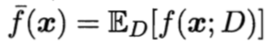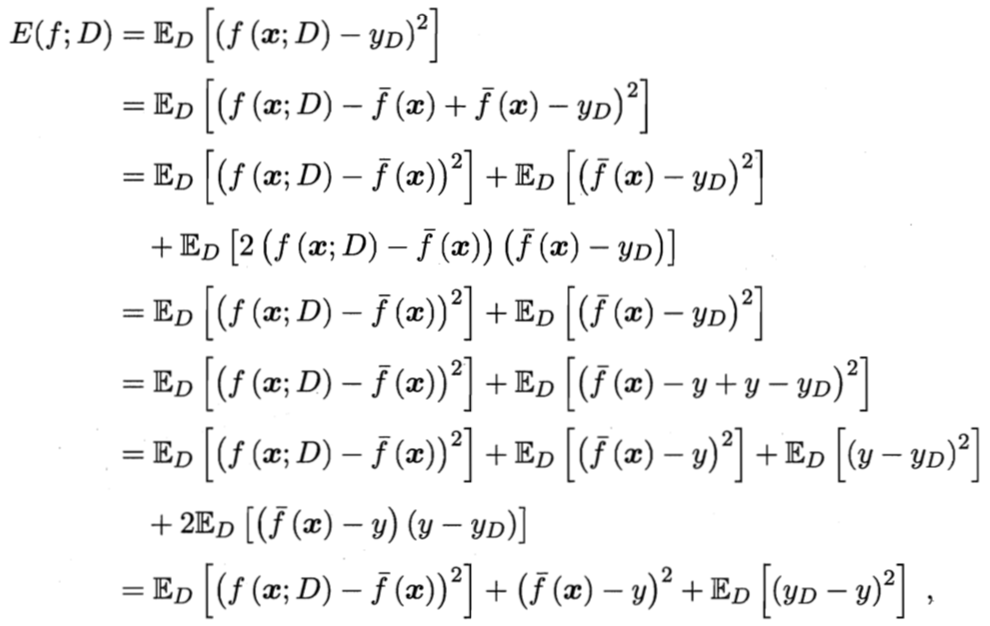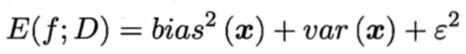## 0x1：方差：模型的预测稳定性 - 数据扰动对模型的影响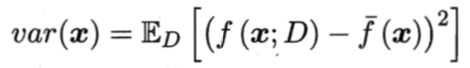1. 控制训练轮数、提前停止训练、学习率逐渐减小渐进逼近等策略；
2. 使用例如决策树里的剪枝策略，控制模型复杂度；
3. 使用正则化等手段，控制模型复杂度；
4. 使用Dropout手段，控制模型复杂度；

## 0x2：噪声：模型在当前任务能达到的期望泛化误差的下届 - 数据决定算法的上界，算法只是在逼近这个上界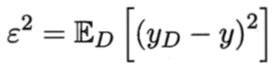## 0x3：偏差：考察模型本身拟合能力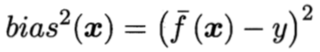## 0x4：偏差-方差窘境（bias-variance dilemma）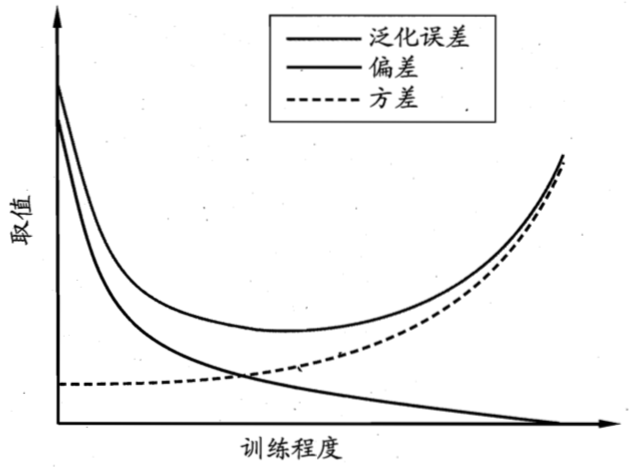# 2. 经验误差（empirical error）与过拟合(overfitting)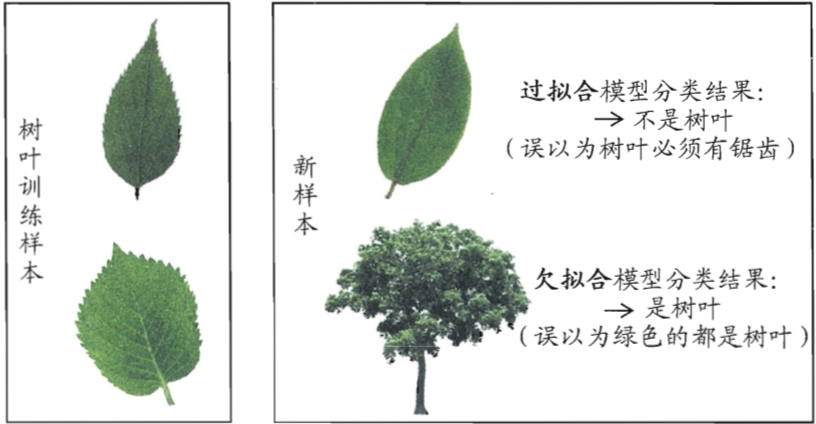## 0x2：什么是过拟合？

### 1. 判断发生过拟合的现象 - 训练集上得到的模型无法适应测试集

#### 1）数据集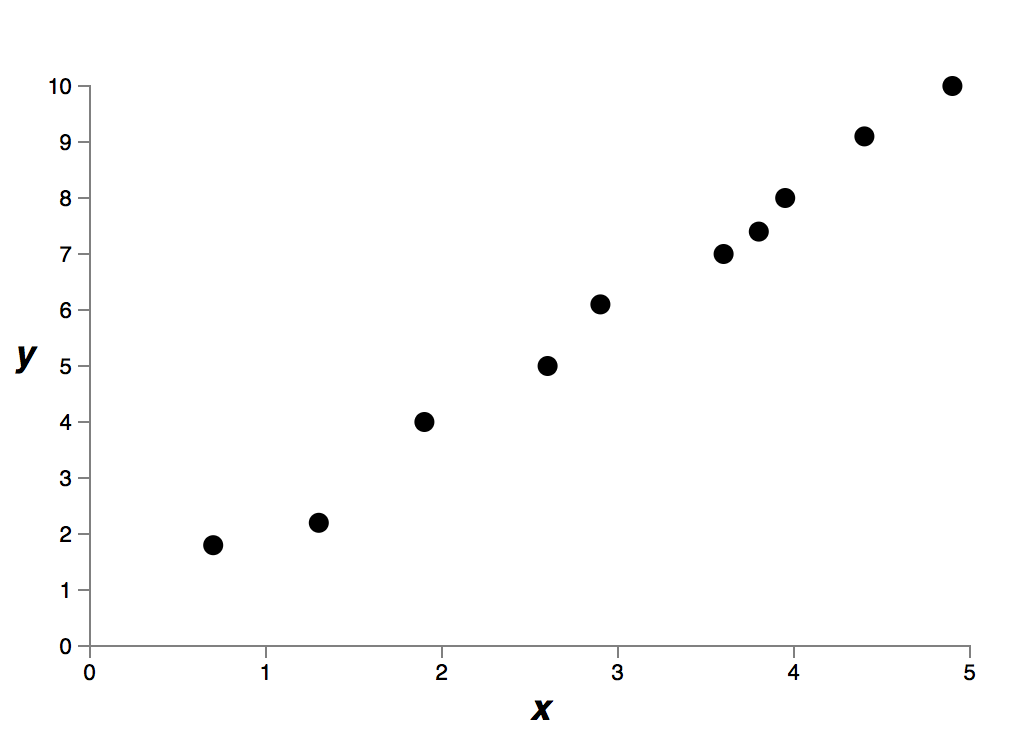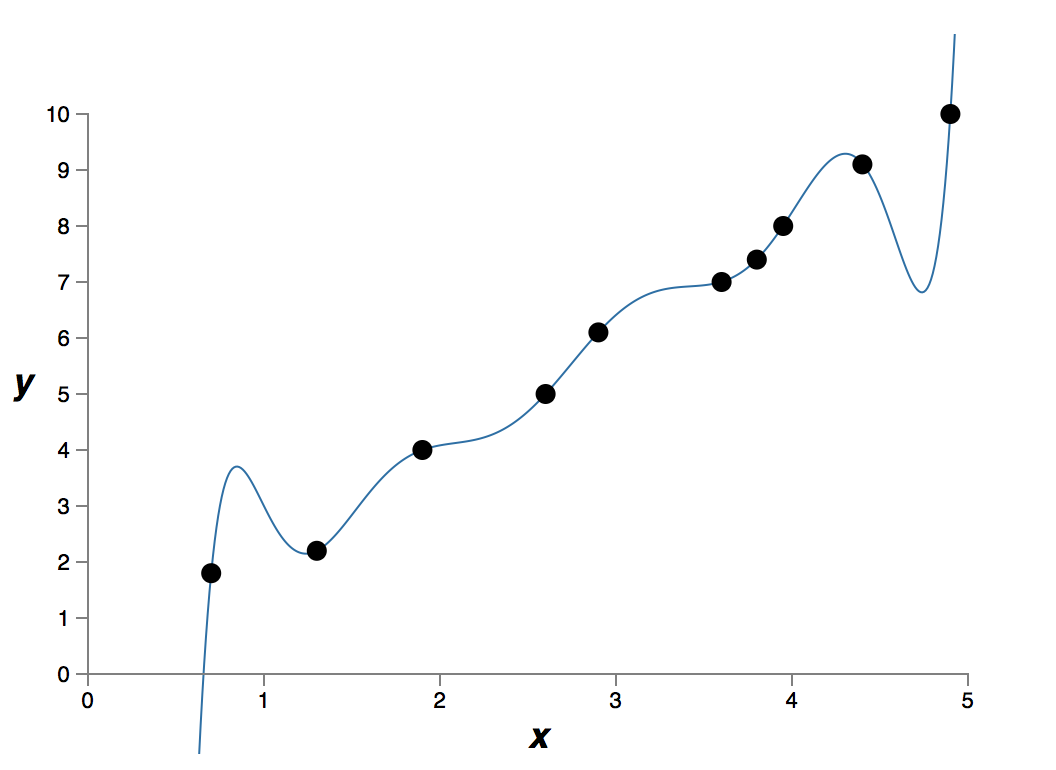#### 3）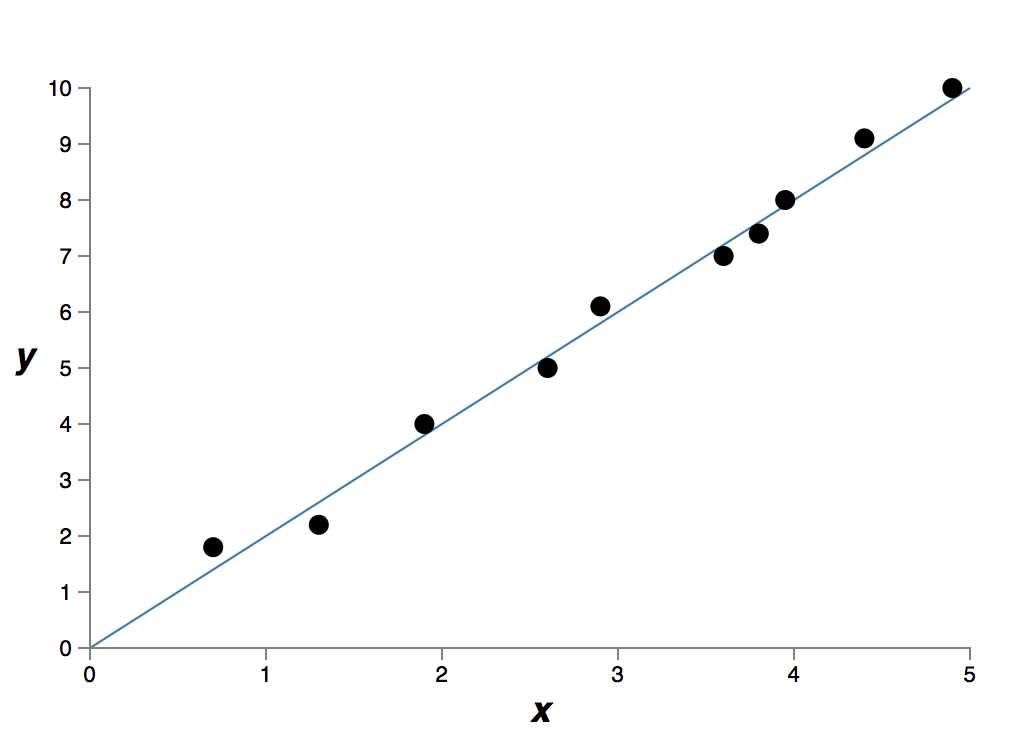### 2. 过拟合产生的本质原因

1. 训练集和测试机特征分布不一致：

2. 在有限的样本中搜索过大的模型空间

3. 训练过程中函数过多吸收了噪音数据的影响
fit model的时候加的parameter太多了，导致model太精准地抓住了这组数据的所有variance，不管是主要的数据趋势带来的variance还是噪音带来的variance都一并被拟合在了模型里。

### 3. 泛化误差评估方法

#### 1. 留出法（hold-out）

1. 若令训练集S包含绝大多数样本，则训练出的模型可能更接近于D训练出的模型，但由于T比较小，评估结果可能不够稳定准确；
2. 若令测试集T多包含一些样本，则训练集S与D的差别更大了，被评估的模型与用D训练出的模型相比可能有较大的差别，从而降低了评估结果的保真性；

#### 3. 自助法（bootstrapping）

1. 给定包含m个样本的数据集D，每次随机从D中随机采集一个样本，并放入D'中，并放回该样本；
2. 这个过程重复m次后，我们就得到了包含m个样本的数据集D’;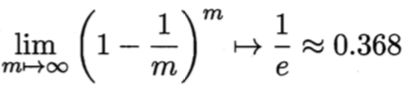estimate）“

## 0x3：复杂函数一定不好吗？复杂函数一定会导致过拟合吗？

### 2. 故事背后的意义

第一，判断两个解释哪个才是真正的「简单」是一个非常微妙的事情；

## 0x4：解决过拟合的一个有效的方法 - 正则化

1. 经典的 bias-variance decomposition；
2. PAC-learning 泛化界解释；
3. Bayes先验解释，这种解释把正则化变成先验

1. 正则化作用一 - 减少权值参数个数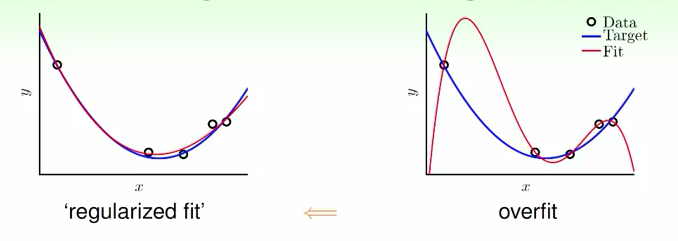2. 正则化作用二 - 降低权值参数数值

https://www.jianshu.com/p/1aafbdf9faa6
https://hit-scir.gitbooks.io/neural-networks-and-deep-learning-zh_cn/content/chap3/c3s5ss2.html
https://www.zhihu.com/question/32246256
https://www.zhihu.com/question/20700829

# 3. 从模型搜索空间限制角度看线性模型中的正则化（Regularization）

## 0x1：正则化简介

L1正则化和L2正则化可以看做是损失函数的惩罚项

## 0x2：Lasso回归 - 包含L1正则化的线性回归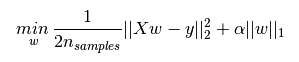，后面一项 α||w||1 即为L1正则化项。||w||1 是指权值向量 w 中各个元素的绝对值之和

### 1. L1正则化的作用

L1正则化可以产生稀疏权值矩阵，即产生一个稀疏模型，可以用于特征选择。

### 2. 以二维损失函数几何角度解释 L1正则化是如何影响模型权重分配的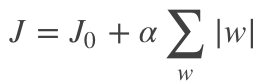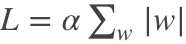，则 J = J0 + L，此时我们的任务变成在 L 约束下求出 J0 取最小值的解。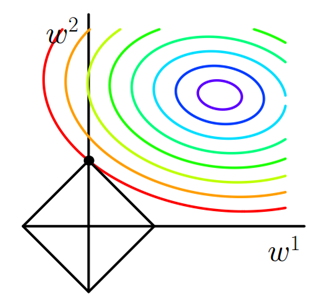α 越小，L 的图形越大（上图中的黑色方框）；
α 越大，L 的图形就越小，可以小到黑色方框只超出原点范围一点点；

1. 使得权重向量 w 尽量稀疏，即被选中的特征尽量少。且 ；
2. 即使被选中，也有能力尽量使得 w 尽量小；

### 3. L1正则化的惩罚因子参数怎么选择

α越大，越容易使得权值向量 w 取得稀疏情况，同时 权值向量 w 值也越小。

## 0x3：Ridge回归 - 包含L2正则化的线性回归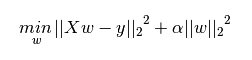，式中加号后面一项 α||w||2 即为L2正则化项。||w||2 是指权值向量 w 中各个元素的平方和然后再求平方根

### 1. L2正则化的作用

L2正则化可以防止模型过拟合（overfitting）。但是这不是L2正则化的专利，L1正则化也能一定程度上防止模型过拟合。

### 2. 以二维损失函数可视化解释 L2正则化是如何使模型权重分配趋向于小值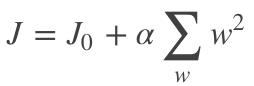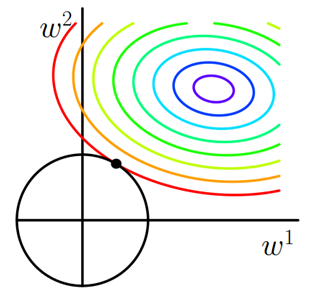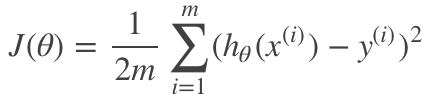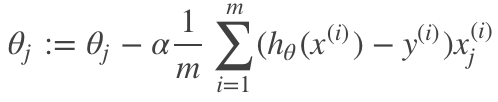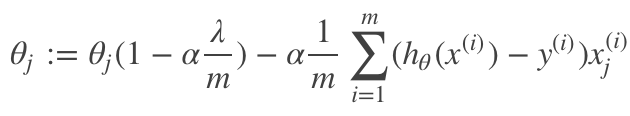与未添加L2正则化的迭代公式相比，每一次迭代，θj 都要先乘以一个小于 1 的因子，从而使得 θj 不断减小，因此总得来看，θ是不断减小的；

### 3. L2正则化的惩罚因子参数怎么选择

λ越大，L2惩罚力度就越大，参数被小值化压制的程度也越大。

## 0x4：L1、L2正则化各自适合的场景

1. ridge regression（L2） 并不具有产生稀疏解的能力，也就是说参数并不会真出现很多零。假设我们的预测结果与两个特征相关，L2正则倾向于综合两者的影响，给影响大的特征赋予高的权重；
2. 而 L1 正则倾向于选择影响较大的参数，而舍弃掉影响较小的那个；

## 0x5：不同惩罚参数下，正则化效果可视化

# -*- coding: utf-8 -*-

from __future__ import print_function
import keras
from keras.datasets import mnist
from keras.models import Sequential, Model
from keras.layers import Dense, Dropout, Flatten, Input
from keras.layers import Conv2D, MaxPooling2D
from keras import backend as K
from keras import initializers
import numpy as np
import matplotlib.pyplot as plt
from keras import regularizers

(x_train, y_train), (x_test, y_test) = mnist.load_data()
x_train = x_train.reshape(x_train.shape, 28, 28, 1)
x_test = x_test.reshape(x_test.shape, 28, 28, 1)
x_train = x_train.astype('float32')
x_test = x_test.astype('float32')
x_train /= 255.0
x_test /= 255.0
y_train = keras.utils.to_categorical(y_train, 10)
y_test = keras.utils.to_categorical(y_test, 10)

def my_reg(weight_matrix):
#return 0    # 无正则化
return 1.0 * K.sum(K.abs(weight_matrix))   # L1正则化
#return 2.0 * K.sum(K.abs(weight_matrix))   # L1正则化
#return 1.0 * K.sum(K.pow(K.abs(weight_matrix), 2))   # L2正则化
#return 2.0 * K.sum(K.pow(K.abs(weight_matrix), 2))   # L2正则化
#return 1.0 * K.sum(K.abs(weight_matrix)) + 1.0 * K.sum(K.pow(K.abs(weight_matrix), 2)) # L1-L2混合正则化
#return K.sum(K.pow(K.abs(weight_matrix), 3))   # L3正则化

# 所有权重初始化为均值0，方差0.5的正态分布
init = initializers.random_normal(mean=0, stddev=0.25, seed=42)

input = Input(shape=(28, 28, 1))
conv1 = Conv2D(32, kernel_size=(3, 3), activation='relu', kernel_initializer=init, kernel_regularizer=my_reg)(input)
conv2 = Conv2D(64, (3, 3), activation='relu', kernel_initializer=init, kernel_regularizer=my_reg)(conv1)
pool1 = MaxPooling2D(pool_size=(2, 2))(conv2)
conv3 = Conv2D(128, (3, 3), activation='relu', kernel_initializer=init, kernel_regularizer=my_reg)(pool1)
pool2 = MaxPooling2D(pool_size=(2, 2))(conv3)
flat = Flatten()(pool2)
dense1 = Dense(128, activation='relu', kernel_initializer=init, kernel_regularizer=my_reg)(flat)
output = Dense(10, activation='softmax', kernel_initializer=init, kernel_regularizer=my_reg)(dense1)
model = Model(inputs=input, outputs=output)
model.summary()

for i in range(40):
model.fit(x_train, y_train, batch_size=128, epochs=1, verbose=0, validation_data=(x_test, y_test))  # 每次只训练一轮
score = model.evaluate(x_test, y_test, verbose=0)
weights = model.get_weights()
all_weights = np.zeros([0, ])
for w in weights:
w_flatten = np.reshape(w, [-1])
all_weights = np.concatenate([all_weights, w_flatten], axis=0)
plt.hist(all_weights, bins=100, color="b", normed=True, range=[-1, 1])
print("epoch=" + str(i) + " loss=%.2f ,acc=%.3f" % (score, score))
plt.title("epoch=" + str(i) + " loss=%.2f ,acc=%.3f" % (score, score))
plt.savefig("mnist_model_weights_hist_%d.png" % (i))
plt.clf()

### 1. 无正则化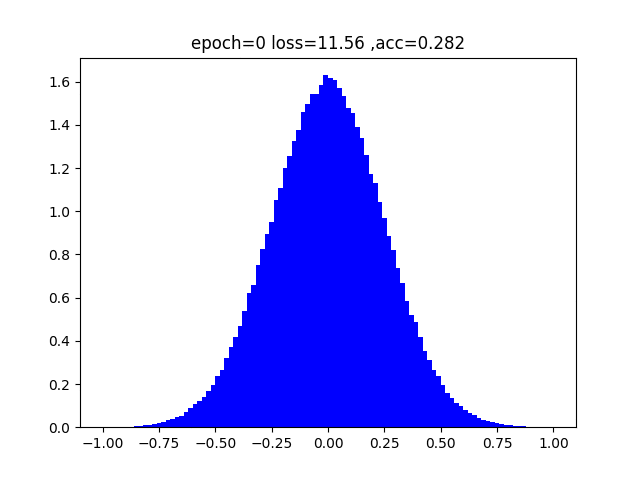### 2. L1正则化 - 惩罚因子 = 1e-4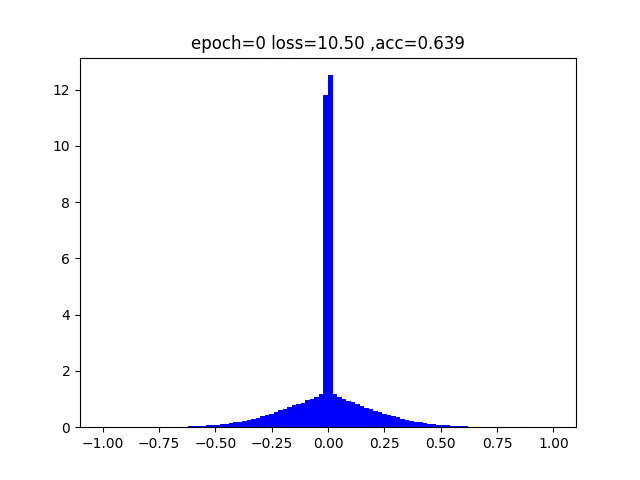### 3. L1正则化 - 惩罚因子 = 1e-3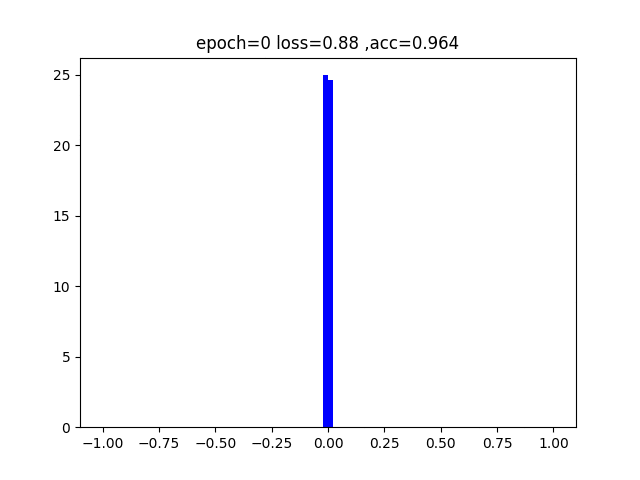### 4. L2正则化 - 惩罚因子 = 1e-4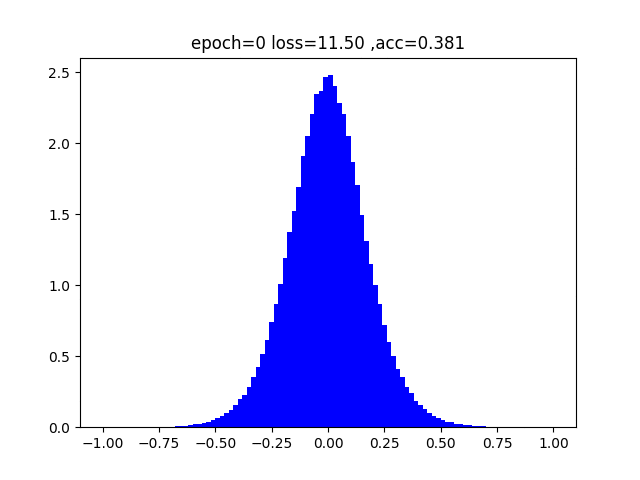### 5. L2正则化 - 惩罚因子 = 1e-3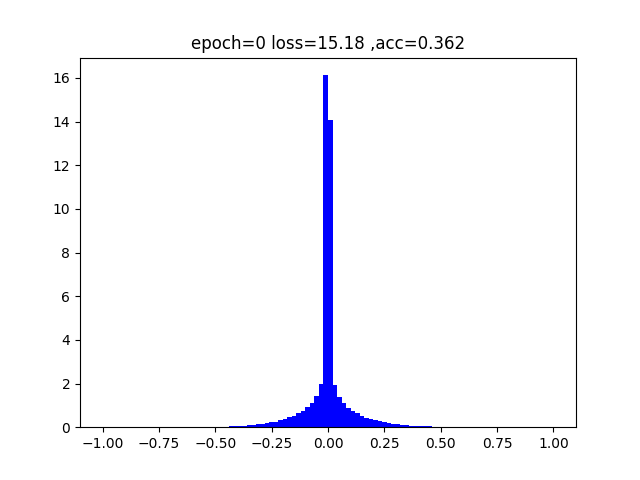### 6. L1-L2混合正则化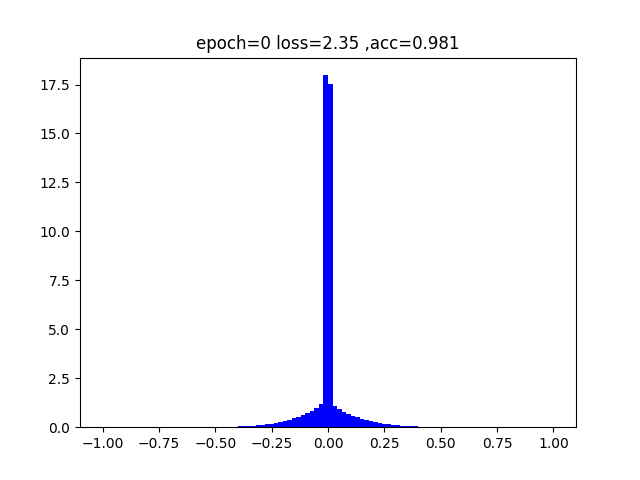### 7. L3正则化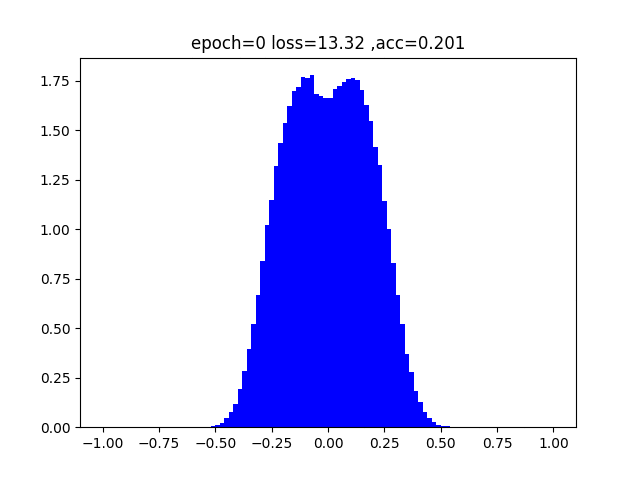## 0x5：从不同正则化参数效果图中我们可以得到什么信息

1. L1/L2正则化都可以将权重往0的方向压制，但是L1的压制效果更强，L2比较慢一些，相同惩罚参数的情况下，L2要比L1花更久的时间达到相同的压制效果。
2. 在保证准确率的前提下，L1/L2正则化能够省下更多的元素，这就意味着模型能够大大地减少其存储空间（将矩阵变成稀疏矩阵的形式保存）
3. 从L1/L2的函数图像来看:
1) 元素绝对值在[0,1]之间的时候，L1对于元素的惩罚更大，L2的惩罚小，相对的容忍度更大;
2) 元素绝对值在[1，∞]之间的时候，L1对于元素的惩罚更小，L2的惩罚大，相对的容忍度更小
4. 从斜率角度来看:
1) L2正则化的斜率大要在[1，∞]才能发挥其功力，在[0,1]之间，往0赶的能力不是很强;
2) 然而L1在所有地方斜率都一样，相对而言，在[0,1]之间，其往0赶的能力强

https://vimsky.com/article/969.html
https://blog.csdn.net/jinping_shi/article/details/52433975
https://blog.csdn.net/yuweiming70/article/details/81513742
https://blog.csdn.net/zouxy09/article/details/24971995
https://blog.csdn.net/yuweiming70/article/details/81513742

# 4. 从贝叶斯估计的角度看线性模型中的正则化

其中正则化项对应后验估计中的先验信息；

## 0x1：不包含正则化的原始线性回归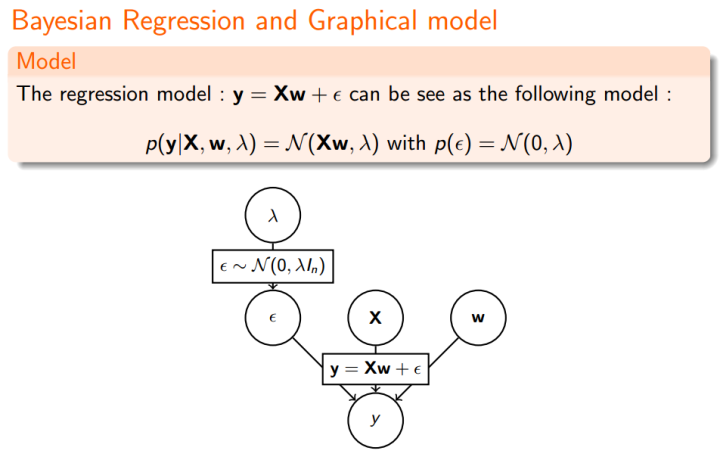### 1. 由最大似然估计(MLE) - 针对不包含正则项的概率分布进行极大似然估计## 0x2：引入高斯分布先验的线性回归 - Ridge Regression。绝对值后面的下标2表示L2范数的意思。

## 0x3：引入拉普拉斯分布(Laplace distribution)先验的线性回归 - LASSO Regression

LASSO - least absolute shrinkage and selection operator.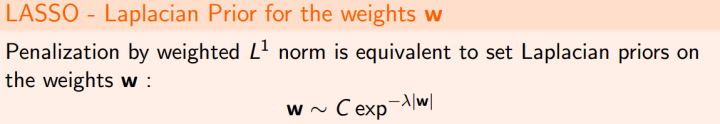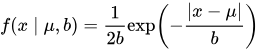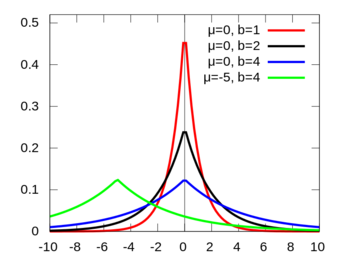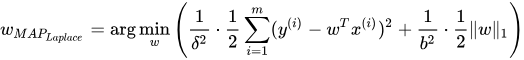。绝对值后面的下标1表示L1范数的意思。

LASSO 仍然是一个 convex optimization（凸优化） 问题，不具有解析解。它的优良性质是能产生稀疏性，导致 w 中许多项变成零。

## 0x4：引入L1-L2先验的线性回归 - Elastic Net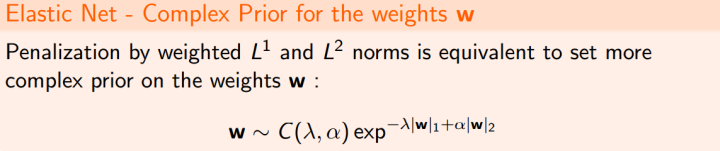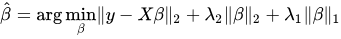。绝对值后面的下标1和下标2表示L1/L2范数的意思。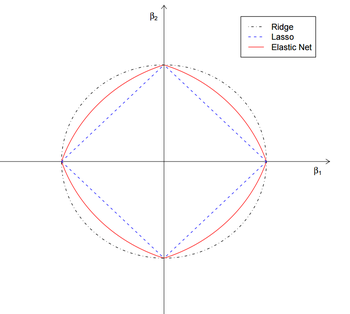https://www.cnblogs.com/LittleHann/p/7309511.html#_label3_4_0_2

https://www.zhihu.com/question/23536142/answer/90135994
https://www.jianshu.com/p/c9bb6f89cfcc
https://blog.csdn.net/zhuxiaodong030/article/details/54408786
http://charleshm.github.io/2016/03/Regularized-Regression/#fn:5
https://ask.julyedu.com/question/150

# 5. 从经验风险/结构风险的角度看线性模型中的正则化

https://www.cnblogs.com/LittleHann/p/7749661.html#_label3_0_2_0

https://blog.csdn.net/zhihua_oba/article/details/78728880

# 6. 非线性模型中的正则化

## 0x1：决策树中的正则化

https://www.cnblogs.com/LittleHann/p/7309511.html

# 7. 从概念描述空间看泛化问题

## 0x1：什么是概念描述空间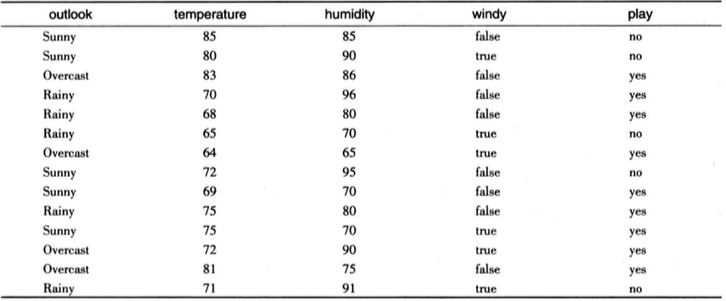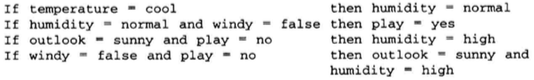• 记忆性规则集：最极端的情况下，对每个样本生成一个对应的规则，这样模型仅仅只是存储和记忆了原始数据集。
• 概括泛化型规则集：最完美的情况下，从所有样本中提取出一个唯一的规则集。这个规则集可以完美概括训练数据集，同时在测试集和未来的未知数据上也能有很好的表现。

## 0x2：训练学习的本质 - 枚举概念空间

### 1. 理想情况下的泛化训练过程

• 一个肯定的样本会去除所有不匹配的描述；
• 而一个否定的样本则会去除所有匹配的描述；

### 2. 真实情况下的训练过程

• 样本在处理后，仍然存在大量的描述，模型不确定熵依然很高
• 或者所有的描述都被去除

• 如果未知的事物与所有剩余的描述集相匹配，就应该将其分到所匹配的目标
• 如果未知的事物不能和其中任何一个相匹配，就把它分到目标概念以外
• 如果未知的事物仅和其中一部分相匹配，就会出现模糊，也就是我们常说的误报和漏报的零和博弈。

## 0x3：从搜索角度看偏差

• 概念描述语言：例如CART4结构或线性回归方程结构
• 在空间中搜索的次序：启发式还是梯度导向式
• 对于特定训练数据避免过度拟合的方法

• 语言偏差（language bias）
• 搜索偏差（search bias）
• 避免过度拟合偏差（overfitting-avoidance bias）

### 2. 搜索偏差

• 采用”贪心（greedy）“搜索策略的搜索：在每一阶段找出最好的规则，然后将其加入规则集。但是问题是，1+1不一定>=2，最好的一对规则并不是单独两个最好规则的简单叠加。
• 构造决策树：最先作出的基于一个特定属性的分割。但是问题是，在后续节点构建时会发现，父节点的选择并不是一个好的决定
• ......

# 8. 缓解过拟合的另一种思路 - 集成学习

• 装袋（bagging）
• 提升（boosting）
• 堆栈（stacking）

## 0x1：装袋（bagging）

• 当对决策树使用装袋技术时，决策树已经是不稳定的，如果不对树进行剪枝，经常可以获得更好的性能，而这会使决策树变得不稳定
• 旋转森林（rotation foreast）的集成学习方法结合了随机子空间和装袋方法，利用主成分特征构建决策树集群。在每一次迭代中，输入属性被随机分类k个不相连的子集，在每个子集中使用主成分分析，以便产生子集中属性的线性组合，即对原始轴的旋转。

## 0x2：提升（boosting）

• 首先，相似点是与装袋法一样，提升利用你投票（用于分类）或者取平均值（用于数值预测）来组合各个模型的输出。
• 另外，也与装袋一样，它组合同一类型的模型，例如决策树。然而，提升是迭代的，在装袋中各个模型是单独建立的，而提升的每个新模型是受先前已建立模型的性能影响的。提升法鼓励新模型成为处理先前模型所不能正确分类的实例的专家。
• 最后一个不同点是，提升根据模型的性能来对每个模型的贡献加权，而不是赋予每个模型相同的权值

posted @ 2018-11-29 19:40  郑瀚Andrew.Hann  阅读(...)  评论(... 编辑 收藏